Function Repository Resource:

# SolveKillerSudokuPuzzle

Solve a killer sudoku puzzle

Contributed by: Paritosh Mokhasi
 ResourceFunction["SolveKillerSudokuPuzzle"][pos,vals] solves a killer sudoku puzzle based on the position pos of cages and the values vals associated with the cages.

## Details and Options

The lengths of the first and second arguments must be the same.
Each element of the first argument must be a list containing the matrix positions of the cage. Each position in a cage represents an unknown number.
Each element of the second argument must be a positive integer and represents the sum of all the numbers in that cage.
The objective is to use the cage information to find all the elements of the board such that the final solved puzzle must obey the three rules of a standard sudoku puzzle.
The three rules of a standard sudoku board are:
First, each row must contain all the numbers 1–9.
Second, each column must contain all the numbers 1–9.
Third, each 3×3 block must contain all the numbers 1–9.

## Examples

### Basic Examples (2)

Solve the following killer sudoku puzzle: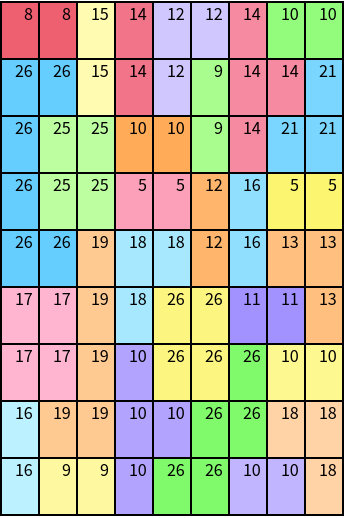In:=Out=Display the solved puzzle:

 In:=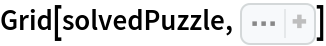Out=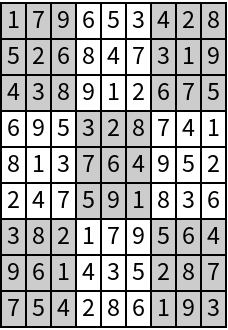### Scope (4)

The cage positions are a List of sublists where each sublist contains the positions within that cage:

 In:=Out=The cage values are the sums of the numbers at each position in the cage:

 In:=Out=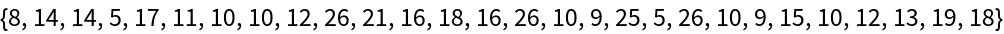Solve the puzzle:

 In:=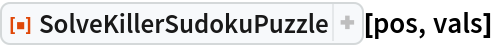Out=See the solution:

 In:=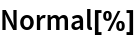Out=Paritosh Mokhasi

## Version History

• 1.0.0 – 19 May 2020